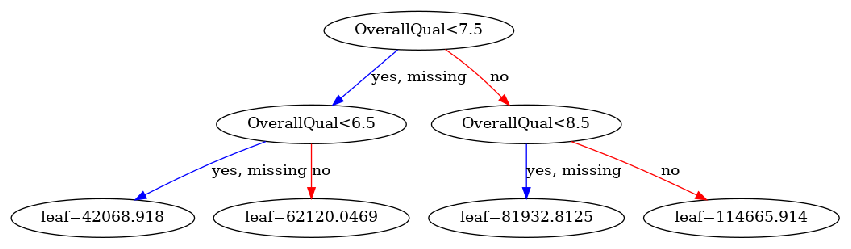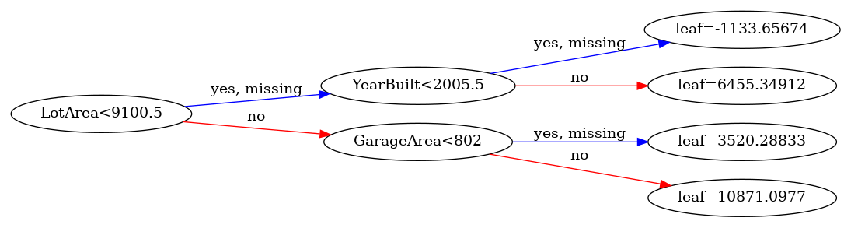import pandas as pd
import numpy as np
import matplotlib.pyplot as plt
import xgboost as xgb

plt.rcParams['figure.figsize'] = (7, 7)


## Regression review

• Common regression metrics
• Root Mean Squared Error (RMSE)
• Mean Absolute Erro (MAE)

## Objective (loss) functions and base learners

• Objective functions and Why we use them
• Quantifies how far off a prediction is from the actual result
• Measures the difference between estimated and true values for some collection of data
• Goal: Find the model that yields the minimum value of the loss function
• Common loss functions and XGBoost
• Loss function names in xgboost:
• reg:linear - use for regression problems
• reg:logistic - use for classification problems when you want just decision, not probability
• binary:logistic - use when you want probability rather than just decision
• Base learners and why we need them
• XGBoost involves creating a meta-model that is composed of many individual models that combine to give a final prediction
• Individual models = base learners
• Want base learners that when combined create final prediction that is non-linear
• Each base learner should be good at distinguishing or predicting different parts of the dataset
• Two kinds of base learners: tree and linear

### Decision trees as base learners

It's now time to build an XGBoost model to predict house prices - not in Boston, Massachusetts, as you saw in the video, but in Ames, Iowa! This dataset of housing prices has been pre-loaded into a DataFrame called df. If you explore it in the Shell, you'll see that there are a variety of features about the house and its location in the city.

In this exercise, your goal is to use trees as base learners. By default, XGBoost uses trees as base learners, so you don't have to specify that you want to use trees here with booster="gbtree".

Note: reg:linear is replaced with reg:squarederror
df = pd.read_csv('./dataset/ames_housing_trimmed_processed.csv')
X, y = df.iloc[:, :-1], df.iloc[:, -1]

from sklearn.model_selection import train_test_split
from sklearn.metrics import mean_squared_error

# Create the training and test sets
X_train, X_test, y_train, y_test = train_test_split(X, y, test_size=0.2, random_state=123)

# Instantiatethe XGBRegressor: xg_reg
xg_reg = xgb.XGBRegressor(objective='reg:squarederror', seed=123, n_estimators=10)

# Fit the regressor to the training set
xg_reg.fit(X_train, y_train)

# Predict the labels of the test set: preds
preds = xg_reg.predict(X_test)

# compute the rmse: rmse
rmse = np.sqrt(mean_squared_error(y_test, preds))
print("RMSE: %f" % (rmse))

RMSE: 28106.463641


### Linear base learners

Now that you've used trees as base models in XGBoost, let's use the other kind of base model that can be used with XGBoost - a linear learner. This model, although not as commonly used in XGBoost, allows you to create a regularized linear regression using XGBoost's powerful learning API. However, because it's uncommon, you have to use XGBoost's own non-scikit-learn compatible functions to build the model, such as xgb.train().

In order to do this you must create the parameter dictionary that describes the kind of booster you want to use (similarly to how you created the dictionary in Chapter 1 when you used xgb.cv()). The key-value pair that defines the booster type (base model) you need is "booster":"gblinear".

Once you've created the model, you can use the .train() and .predict() methods of the model just like you've done in the past.

DM_train = xgb.DMatrix(data=X_train, label=y_train)
DM_test = xgb.DMatrix(data=X_test, label=y_test)

# Create the parameter dictionary: params
params = {"booster":"gblinear", "objective":"reg:squarederror"}

# Train the model: xg_reg
xg_reg = xgb.train(params=params, dtrain=DM_train, num_boost_round=5)

# Predict the labels of the test set: preds
preds = xg_reg.predict(DM_test)

# Compute and print the RMSE
rmse = np.sqrt(mean_squared_error(y_test, preds))
print("RMSE: %f" % (rmse))

RMSE: 45098.309059


### Evaluating model quality

It's now time to begin evaluating model quality.

Here, you will compare the RMSE and MAE of a cross-validated XGBoost model on the Ames housing data.

housing_dmatrix = xgb.DMatrix(data=X, label=y)

# Create the parameter dictionary: params
params = {"objective":"reg:squarederror", "max_depth":4}

# Perform cross-valdiation: cv_results
cv_results = xgb.cv(dtrain=housing_dmatrix, params=params, nfold=4,
num_boost_round=5, metrics='rmse', as_pandas=True, seed=123)

# Print cv_results
print(cv_results)

# Extract and print final boosting round metric
print((cv_results['test-rmse-mean']).tail(1))

   train-rmse-mean  train-rmse-std  test-rmse-mean  test-rmse-std
0    141767.531250      429.454591   142980.433594    1193.798509
1    102832.546875      322.475723   104891.396485    1223.159762
2     75872.615234      266.472656    79478.937500    1601.344539
3     57245.652344      273.624748    62411.920899    2220.150028
4     44401.296875      316.425549    51348.278320    2963.377786
4    51348.27832
Name: test-rmse-mean, dtype: float64

housing_dmatrix = xgb.DMatrix(data=X, label=y)

# Create the parameter dictionary: params
params = {"objective":"reg:squarederror", "max_depth":4}

# Perform cross-valdiation: cv_results
cv_results = xgb.cv(dtrain=housing_dmatrix, params=params, nfold=4,
num_boost_round=5, metrics='mae', as_pandas=True, seed=123)

# Print cv_results
print(cv_results)

# Extract and print final boosting round metric
print((cv_results['test-mae-mean']).tail(1))

   train-mae-mean  train-mae-std  test-mae-mean  test-mae-std
0   127343.462890     668.331024  127633.988281   2404.014089
1    89770.056640     456.961189   90122.503906   2107.909888
2    63580.789062     263.409865   64278.559571   1887.569709
3    45633.156250     151.883825   46819.168945   1459.817731
4    33587.090820      87.000638   35670.646484   1140.607452
4    35670.646484
Name: test-mae-mean, dtype: float64


## Regularization and base learners in XGBoost

• Regularization in XGBoost
• Regularization is a control on model complexity
• Want models that are both accurate and as simple as possible
• Regularization parameters in XGBoost:
• Gamma - minimum loss reduction allowed for a split to occur
• alpha - L1 regularization on leaf weights, larger values mean more regularization
• lambda - L2 regularization on leaf weights
• Base learners in XGBoost
• Linear Base learner
• Sum of linear terms
• Boosted model is weighted sum of linear models (thus is itself linear)
• Rarely used
• Tree Base learner
• Decision tree
• Boosted model is weighted sum of decision trees (nonlinear)
• Almost exclusively used in XGBoost

### Using regularization in XGBoost

Having seen an example of l1 regularization in the video, you'll now vary the l2 regularization penalty - also known as "lambda" - and see its effect on overall model performance on the Ames housing dataset.

housing_dmatrix = xgb.DMatrix(data=X, label=y)

reg_params = [1, 10, 100]

# Create the initial parameter dictionary for varying l2 strength: params
params = {"objective":"reg:squarederror", "max_depth":3}

# Create an empty list for storing rmses as a function of l2 complexity
rmses_l2 = []

# Iterate over reg_params
for reg in reg_params:
# Update l2 strength
params['lambda'] = reg

# Pass this updated param dictionary into cv
cv_results_rmse = xgb.cv(dtrain=housing_dmatrix, params=params, nfold=2,
num_boost_round=5, metrics='rmse', as_pandas=True, seed=123)

# Append best rmse (final round) to rmses_l2
rmses_l2.append(cv_results_rmse['test-rmse-mean'].tail(1).values)

# Loot at best rmse per l2 param
print("Best rmse as a function of l2:")
print(pd.DataFrame(list(zip(reg_params, rmses_l2)), columns=["l2", "rmse"]))

Best rmse as a function of l2:
l2          rmse
0    1  52275.355468
1   10  57746.062500
2  100  76624.625000


### Visualizing individual XGBoost trees

Now that you've used XGBoost to both build and evaluate regression as well as classification models, you should get a handle on how to visually explore your models. Here, you will visualize individual trees from the fully boosted model that XGBoost creates using the entire housing dataset.

XGBoost has a plot_tree() function that makes this type of visualization easy. Once you train a model using the XGBoost learning API, you can pass it to the plot_tree() function along with the number of trees you want to plot using the num_trees argument.

housing_dmatrix = xgb.DMatrix(data=X, label=y)

# Create the parameters dictionary: params
params = {"objective":'reg:squarederror', 'max_depth':2}

# Train the model: xg_reg
xg_reg = xgb.train(dtrain=housing_dmatrix, params=params, num_boost_round=10)

# Plot the first tree
fig, ax = plt.subplots(figsize=(15, 15))
xgb.plot_tree(xg_reg, num_trees=0, ax=ax);

# Plot the fifth tree
fig, ax = plt.subplots(figsize=(15, 15))
xgb.plot_tree(xg_reg, num_trees=4, ax=ax);

# Plot the last tree sideways
fig, ax = plt.subplots(figsize=(15, 15))
xgb.plot_tree(xg_reg, rankdir="LR", num_trees=9, ax=ax);Have a look at each of the plots. They provide insight into how the model arrived at its final decisions and what splits it made to arrive at those decisions. This allows us to identify which features are the most important in determining house price. In the next exercise, you'll learn another way of visualizing feature importances.

### Visualizing feature importances: What features are most important in my dataset

Another way to visualize your XGBoost models is to examine the importance of each feature column in the original dataset within the model.

One simple way of doing this involves counting the number of times each feature is split on across all boosting rounds (trees) in the model, and then visualizing the result as a bar graph, with the features ordered according to how many times they appear. XGBoost has a plot_importance() function that allows you to do exactly this, and you'll get a chance to use it in this exercise!

housing_dmatrix = xgb.DMatrix(data=X, label=y)

# Create the parameter dictionary: params
params = {"objective":"reg:squarederror", "max_depth":4}

# Train the model: xg_reg
xg_reg = xgb.train(dtrain=housing_dmatrix, params=params, num_boost_round=10)

# Plot the feature importance
xgb.plot_importance(xg_reg);
plt.tight_layout()